# Area Of Trapezium

Area of trapezium is the region covered by a trapezium in a two-dimensional plane. It is the space enclosed in 2D geometry. A trapezium is a 2D shape which falls under category of quadrilaterals. Similar to other geometrical shapes, it also has its own properties and formulas based on area and perimeter. Let us learn in detail.

## Trapezium Definition

A  trapezium is a quadrilateral, which is defined as a shape with four sides, which has one set of

parallel sides.  The trapezium is basically a types of quadrilaterals, with exactly one pair of parallel sides. Apart from Trapezium, there are four more types of quadrilaterals, such as;

1. Parallelogram
2. Rectangle
3. Square
4. Rhombus

All these quadrilaterals have one common property, which is, the sum of all the angles is 3600

### Trapezium Basic Concept

1. The pair of parallel sides are called the bases while the non-parallel sides are called the legs of the trapezoid
2. The line segment connecting the midpoints of the non-parallel sides of a trapezoid is called the mid-segment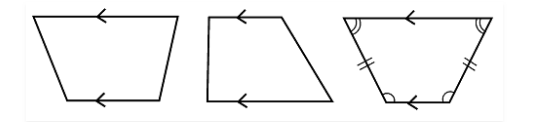3. Check above the different types of trapezium figures, where arrow represents the parallel side of it. In all the three figures you can see, the two sides are parallel to each other, whereas the other two sides are non-parallel
4. If we draw a line segment, between the two non-parallel sides, from the centre of both sides,  the trapezium will be divided into two unequal parts.
5. You must have learned of isosceles triangles, where the two sides of a triangle are equal and the angle opposite the equal sides are also equal. In the same way, we have a figure, which is stated as Isosceles Trapezium, where the two non-parallel sides are equal and form equal angles at one of the bases. You can see the example of it, in the third figure given above.

## Properties of a Trapezium

Here, we are going to learn about some more properties of the trapezium, which is also called as a trapezoid. A trapezium has the following properties:

1. Like other quadrilaterals, the sum of all the four angles of the trapezium is equal to 3600
2. A Trapezium has 4 unequal sides
3. A Trapezium has two parallel sides and two non-parallel sides
4. The diagonals of trapezium bisect each other
5. The length of the mid-segment is equal to 1/2 the sum of the parallel bases, in a trapezium
6. No sides, angles and diagonals of the trapezium are congruent

## Area of a Trapezium

Trapezium area can be calculated by using the below formula:

• Area = (1/2) h (AB+CD)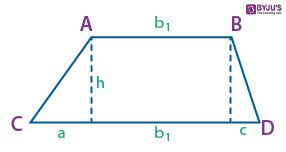### Perimeter of Trapezium

The perimeter of trapezium formula is given by:

• Perimeter = Sum of all the sides =  AB + BC + CD + DA## Derivation of Area of a Trapezium

Following is the derivation for computing the area of the trapezium:

The area of a trapezoid is equal to the sum of the areas  of the two triangles and the area  of the rectangle.

We know that

area of trapezoid  = area of triangle 1 + area of rectangle + area of triangle 2.

which means that.

Substituting the values we have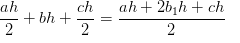Simplifying the equation, rearranging the terms, and factoring result to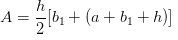If we let the longer base of the trapezoid be b2

then,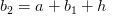Substituting we have,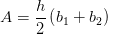Therefore the area of a trapezoid with bases b1 , b2 and altitude h is,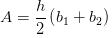### Applications of Trapezium

The concept is a highly used concept in various physics computations and other mathematical calculations. This is the basis for obtaining the equations of motion as described in the 9th CBSE science textbook. The blend of the physics equations and mathematical calculations is very well explained to clear the level of understanding of a budding engineering mind.

For the complete understanding and concept clearance from the BYJU’S one must go through the practice worksheets to check their hands on the concept and test learning.

1. Nafees Malik

How the diagonals of a trapezium bisect each other?

1. balaji_byjus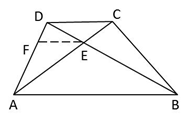ABCD is a trapezium.
Draw EF || BA || CD, intersecting AD in F.
FE || AB (from figure)
DE/EB = DF/FA [basic proportionality theorem] … (1)
FE || DC (from figure)
CE/EA = DF/FA [basic proportionality theorem] …(2)
From equations (1) and (2), we get
DE/EB = CE/EA
i.e., diagonals of a trapezium divide each other proportionally.
Therefore, AC and BD bisect each other
Hence, we conclude that,
Diagonals of a trapezium bisect each other